# scales 1.2.0

We’re very pleased to announce the release of scales 1.2.0. The scales package provides much of the infrastructure that underlies ggplot2’s scales, and using it allow you to customize the transformations, breaks, and labels used by ggplot2. You can install it from CRAN with:

install.packages("scales")

This blog post will show off a few new features for labeling numbers, log scales, and currencies. You can see a full list of changes in the release notes.

library(ggplot2)
library(scales)

## Numbers

label_number() is the workhorse that powers ggplot2’s formatting of numbers, including label_dollar() and label_comma(). This release added a number of useful new features.

The most important is a new scale_cut argument that makes it possible to independently scales different parts of the range. This is useful for scales which span multiple orders of magnitude. Take the following two examples which don’t get great labels by default:

df1 <- data.frame(
x = 10 ^ runif(1000, 2, 9),
y = runif(1000)
)
df2 <- df1 |> dplyr::filter(x <= 1.25 * 10^6)

plot1 <- ggplot(df1, aes(x, y)) +
geom_point() +
labs(x = NULL, y = NULL)
plot1 + scale_x_log10()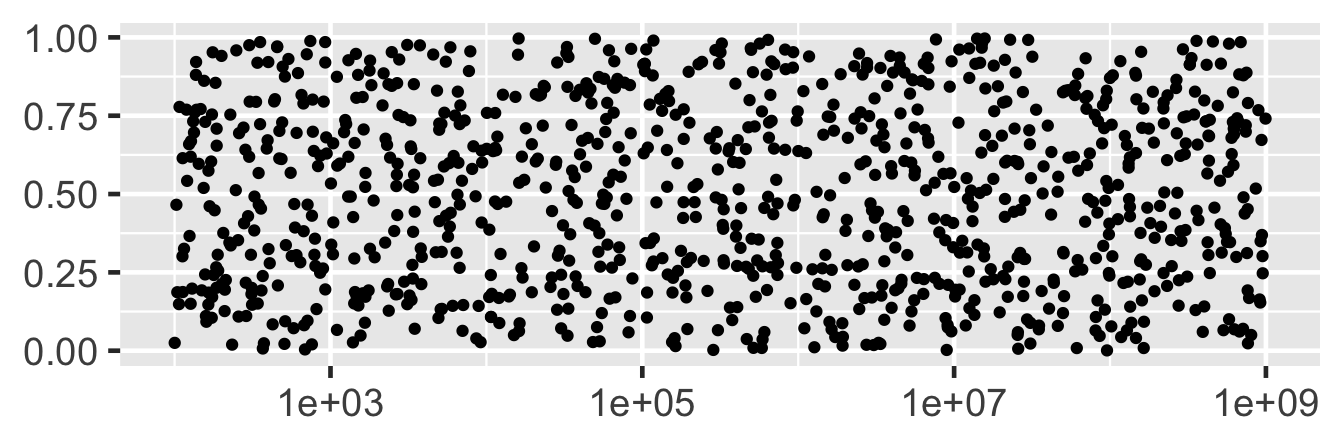plot2 <- ggplot(df2, aes(x, y)) +
geom_point()+
labs(x = NULL, y = NULL)
plot2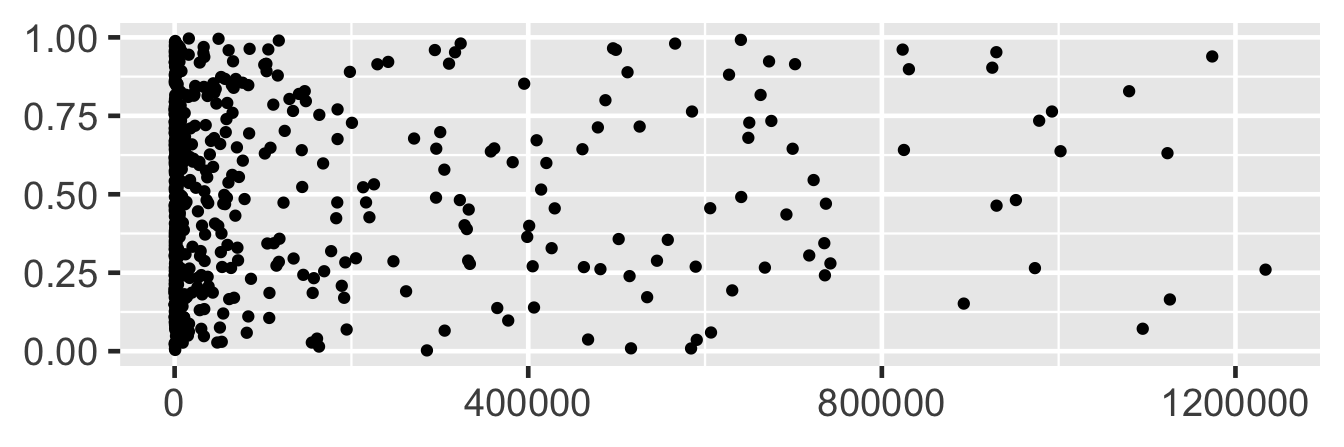You can use cut_short_scale() to show thousands with a K suffix, millions with a M suffix, and billions with a B suffix:

plot1 +
scale_x_log10(
labels = label_number(scale_cut = cut_short_scale())
)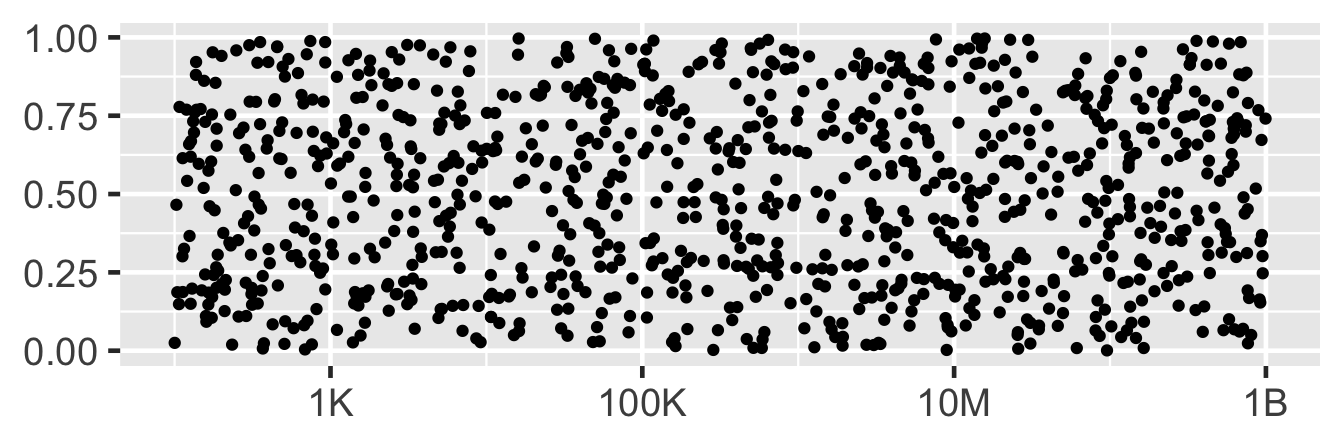plot2 +
scale_x_continuous(
labels = label_number(scale_cut = cut_short_scale())
)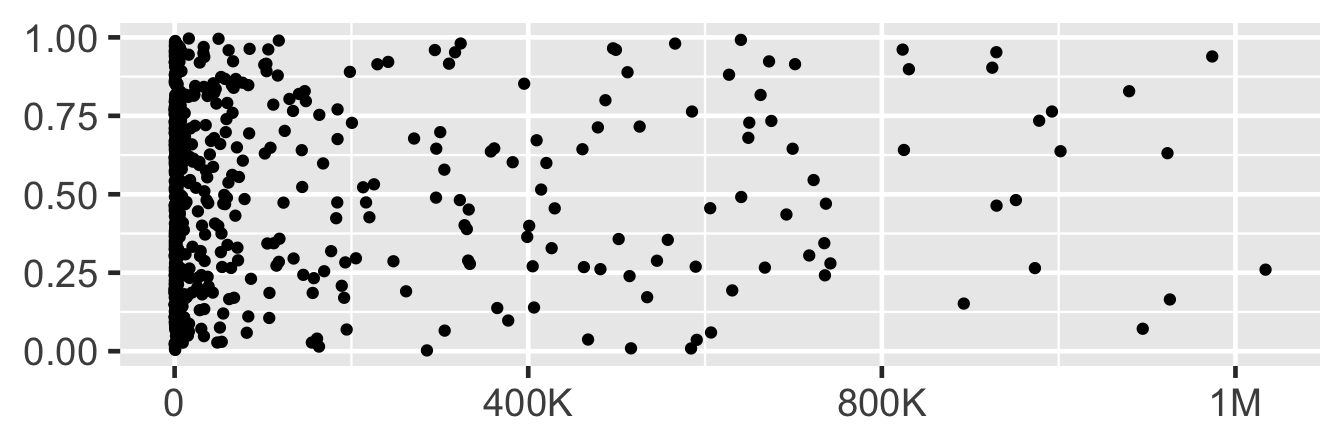(If your country uses 1 billion to mean 1 million million, then you can use cut_long_scale() instead of cut_short_scale().)

You can use cut_si() for SI labels:

plot1 +
scale_x_log10(
labels = label_number(scale_cut = cut_si("g"))
)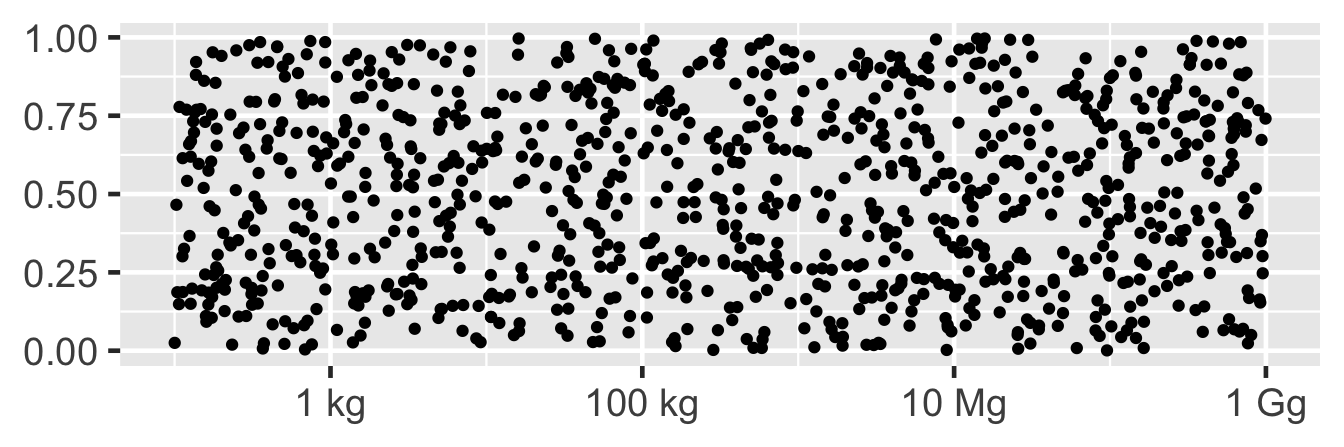plot2 +
scale_x_continuous(
labels = label_number(scale_cut = cut_si("Hz"))
)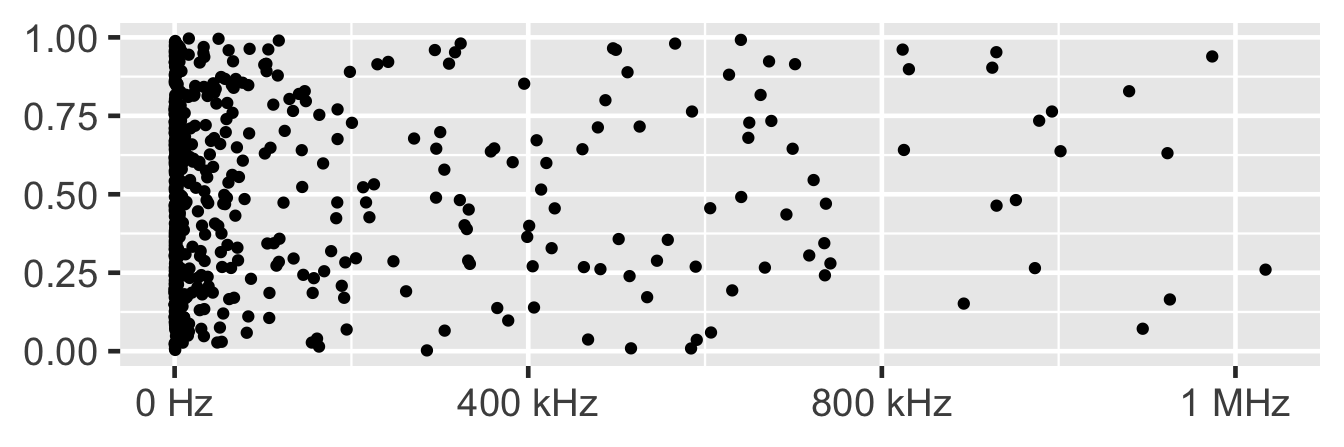This replaces label_number_si() because it incorrectly used the short-scale abbreviations instead of the correct SI prefixes.

## Log labels

Another way to label logs scales, thanks to David C Hall, you can now use scales::label_log() to display

plot1 +
scale_x_log10(
labels = scales::label_log()
)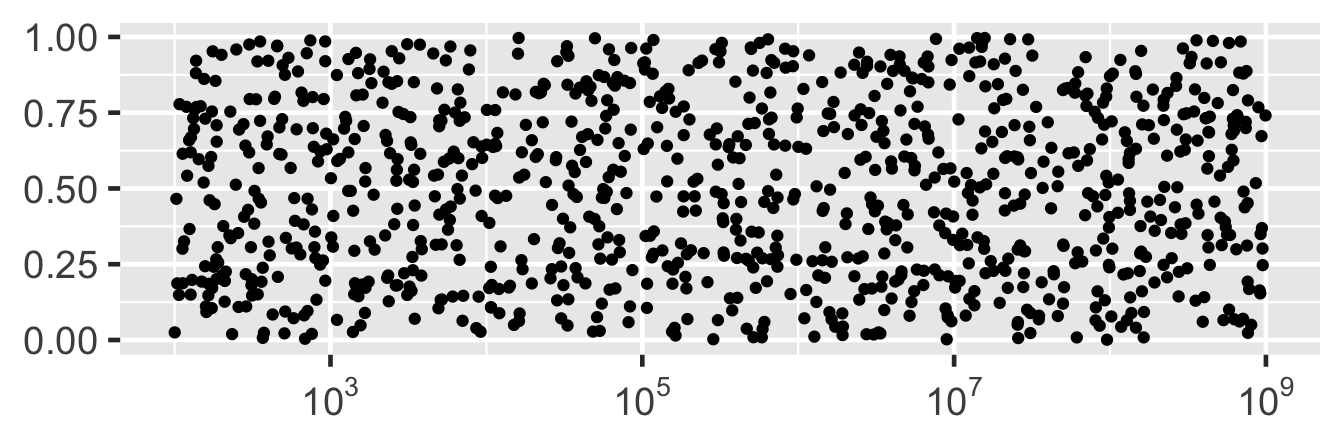You can use the base argument if you need a different base for the a logarithm:

plot1 +
scale_x_continuous(
trans = scales::log_trans(2),
labels = scales::label_log(2)
)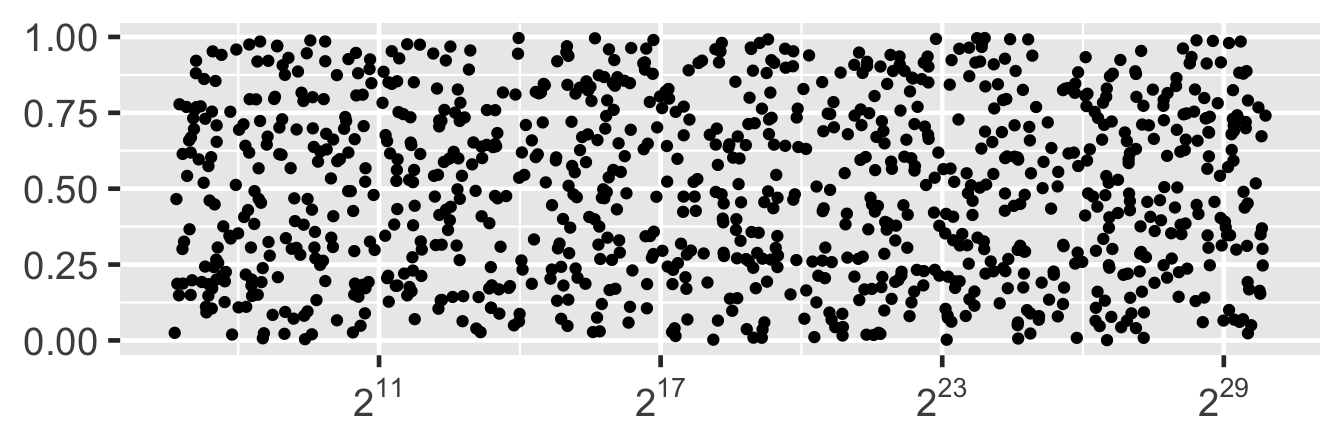## Currency

Finally, label_dollar() receives a couple of small improvements. The prefix is now placed before the negative sign, rather than after it, yielding (e.g) the correct -$1 instead of $-1:

df3 <- data.frame(
date = as.Date("2022-01-01") + 1:1e3,
balance = cumsum(runif(1e3, -1e3, 1e3))
)

plot3 <- ggplot(df3, aes(date, balance)) +
geom_line() +
labs(x = NULL, y = NULL)
plot3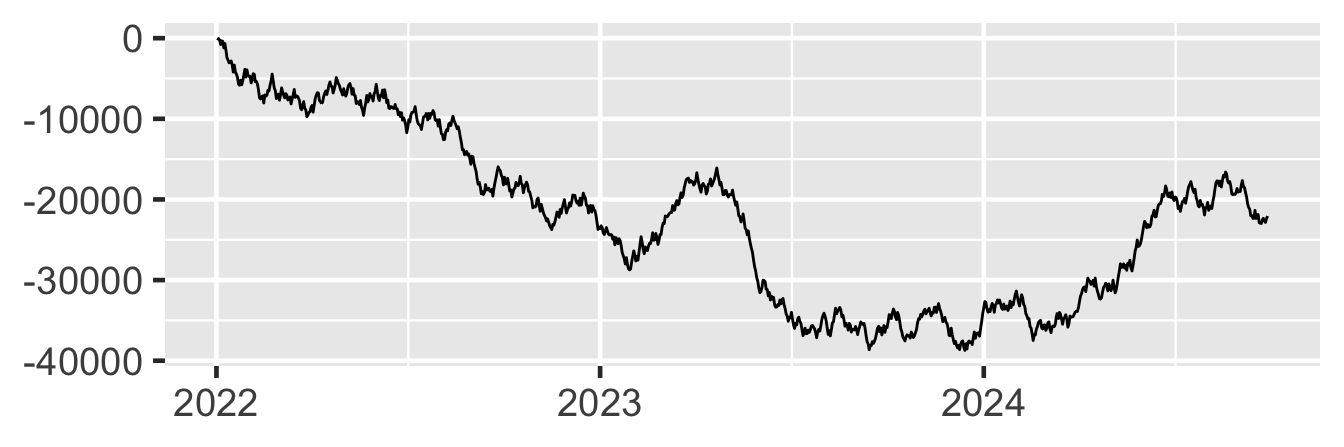plot3 +
scale_y_continuous(
labels = label_dollar(scale_cut = cut_short_scale())
)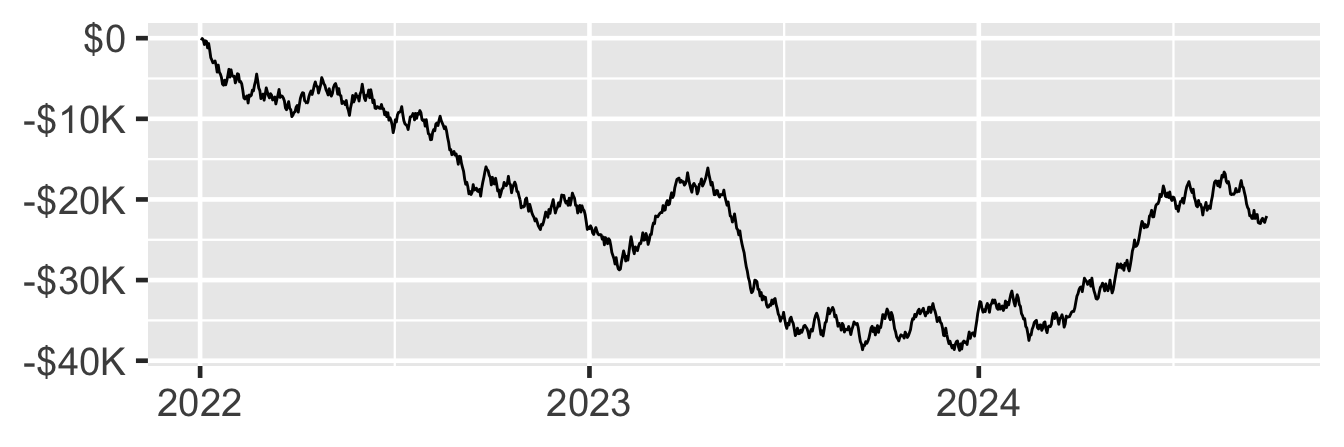It also no longer uses its own negative_parens argument, but instead inherits the new style_negative argument from label_number():

plot3 +
scale_y_continuous(
labels = label_dollar(
scale_cut = cut_short_scale(),
style_negative = "parens"
)
)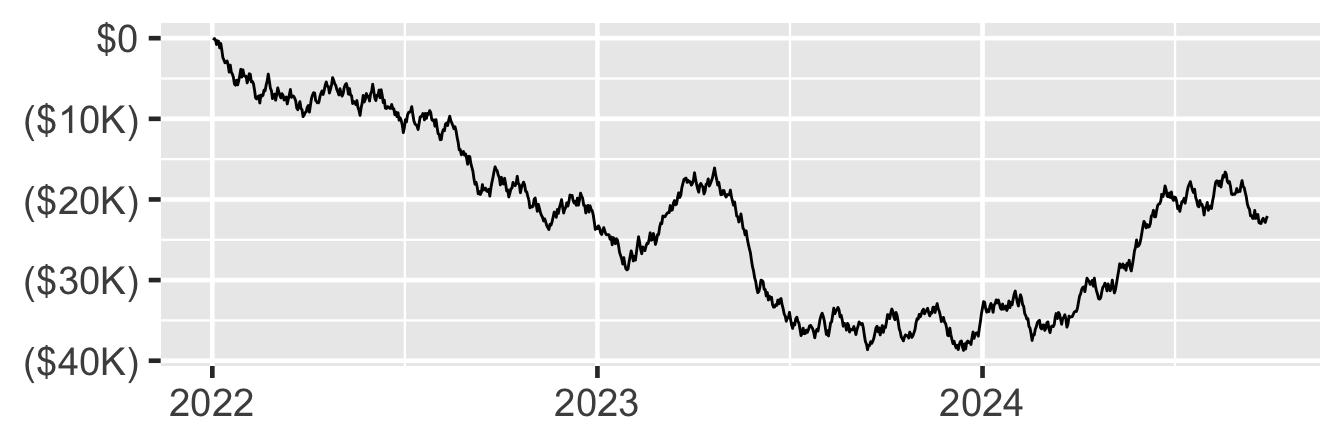## Acknowledgements

A big thanks goes to David C Hall, who contributed to the majority of new features in this version. 40 others contributed by asking questions, identifying bugs, and suggesting patches: @aalucaci, @adamkemberling, @akonkel-aek, @billdenney, @brunocarlin, @campbead, @cawthm, @DanChaltiel, @davidhodge931, @davidski, @dkahle, @donboyd5, @dpseidel, @ds-jim, @EBukin, @elong0527, @eutwt, @ewenme, @fontikar, @frederikziebell, @hadley, @IndrajeetPatil, @jennybc, @karawoo, @mfherman, @mikmart, @mine-cetinkaya-rundel, @mjskay, @nicolaspayette, @NunoSempere, @SimonDedman, @sjackman, @stragu, @teunbrand, @thomasp85, @TonyLadson, @tuoheyd, @vinhtantran, @vsocrates, and @yutannihilation.

Contents